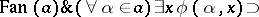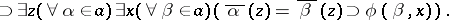# Fan

(diff) ← Older revision | Latest revision (diff) | Newer revision → (diff)

A spread (cf. Spread (in intuitionistic logic))such that for any node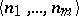ofthere exists only a finite number of natural numbersfor which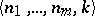is a node of.
In the language of formal intuitionistic mathematical analysis, the function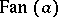, which expresses the concept "the function a defines a fan" , is written as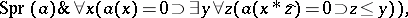(*)
where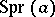means "the function a defines a spread" .
Brouwer's fan theorem: If there exists a rule with the aid of which a certain object — say, a natural number — can be assigned to each element of a fan, then there exists a natural numbersuch that for each element of the fan this object is defined by the firstvalues of the element. Brouwer's theorem is used in proving many specifically intuitionistic facts such as the uniform continuity of every real function defined on a closed interval. In formal intuitionistic mathematical analysis Brouwer's fan theorem is usually proved with the aid of bar induction and Brouwer's continuity principle (cf. Intuitionism). In the language of this formal theory the fan theorem may be written down as follows: